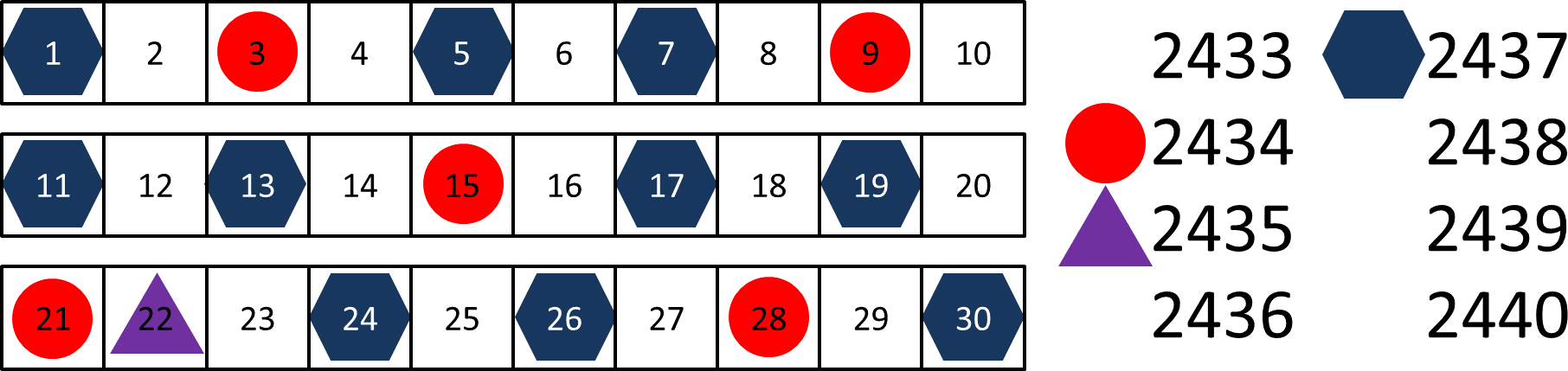# 混合型号排序–复杂实例 排序1

## 对第一个型号排序${ S_k =\frac{\sum_{m=1}^{n} Q_m }{Q_k} }$${ S_{2437} =\frac{17 732 }{5500 } = 3.22}$

## 对第二个型号排序${ S_{2434} =\frac{17 732 }{2766 } = 6.41}$## 对第三个型号排序${ S_{2435} =\frac{17 732 }{121 } = 146.55}$P.S.非常感谢Mark Warren的意见。

## 系列文章

Translated by Xie Xuan